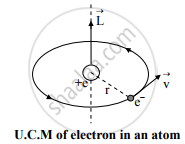# Obtain an Expression for the Magnitude of Magnetic Moment of a Revolving Electron - Physics

In a hydrogen atom, an electron carrying charge 'e' revolves in an orbit of radius 'r' with speed 'v'. Obtain an expression for the magnitude of magnetic moment of a revolving electron.

#### Solution

Expression for magnetic dipole moment:

a. Consider an electron of mass me and charge e revolves in a circular orbit of radius r around the positive nucleus in anticlockwise direction, leading to a clockwise current.b. The angular momentum of an electron due to its orbital motion is given by,
L0 = mevr ….(i)
c. For the sense of orbital motion of electron shown in the figure, the angular momentum vector L acts along normal to the plane of the electron orbit and in  upward direction.
d. Suppose that the period of orbital motion of the electron is T. Then the electron crosses any point on its orbit after every T seconds or 1/T times in one second.
e. Magnitude of circulating current is given by,

I=e(1/T)

But , T=(2pir)/v

I=e(1/((2pir)/v))=(ev)/(2pir)

f. The magnetic dipole moment associated with circulating current is given by,

M_0=IA=(ev)/(2pir)xxpir^2

[∵ Area of current loop, A = πr2]

therefore M_0=(evr)/2     ..........(ii)

This is the required expression.

Concept: Current Loop as a Magnetic Dipole and Its Magnetic Dipole Moment
Is there an error in this question or solution?